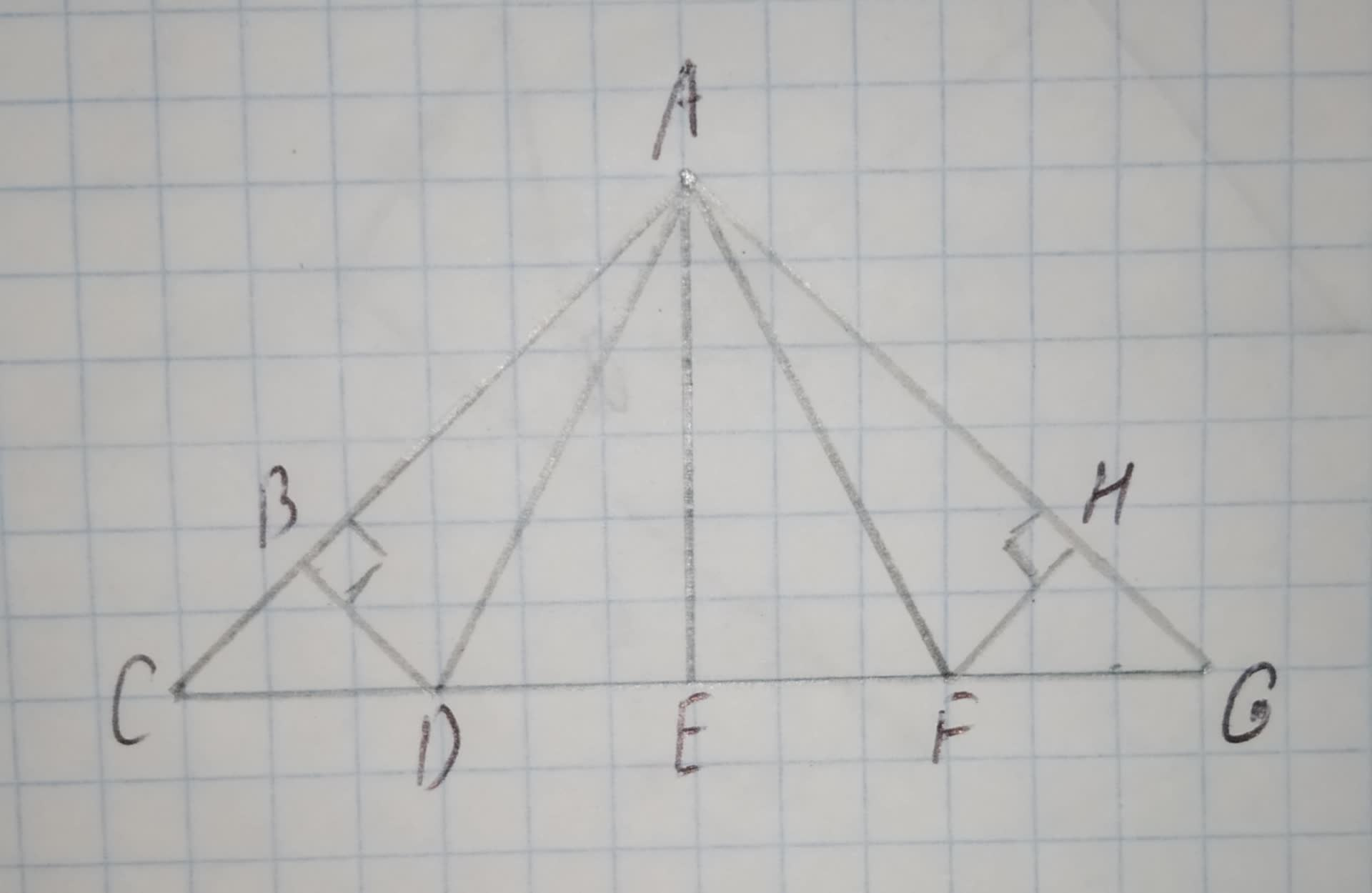# In the roof truss shown, AB = 8 and m/_HAF = 37^(circ). Find: m/_BAD. (HINT: The design of the roof truss displays line symmetry.) 12210203661.jpgCaelan 2021-01-19 Answered
In the roof truss shown, AB = 8 and $m\mathrm{\angle }HAF={37}^{\circ }$. Find: $m\mathrm{\angle }BAD$.
(HINT: The design of the roof truss displays line symmetry.)You can still ask an expert for help

## Want to know more about Congruence?

• Questions are typically answered in as fast as 30 minutes

Solve your problem for the price of one coffee

• Math expert for every subject
• Pay only if we can solve itbahaistag

Find the value of $m\mathrm{\angle }BAD$.
Use transitive property of congruence.
$\mathrm{△}ABD\stackrel{\sim }{=}\mathrm{△}AHF,m\mathrm{\angle }BAD\phantom{\rule{1em}{0ex}}\text{and}\phantom{\rule{1em}{0ex}}m\mathrm{\angle }HAF$ are the angles of congruent triangles, $m\mathrm{\angle }BAD=m\mathrm{\angle }HAF$.
Substitute $m\mathrm{\angle }HAF={37}^{\circ }\in m\mathrm{\angle }BAD=m\mathrm{\angle }HAF$.
$m\mathrm{\angle }BAD={37}^{\circ }$
Therefore, the value of .